# B006 – Calculated Gas Standard Density

## Description

This block calculates the uncertainty in gas standard density determined by the selected calculation method.

## Options

#### Reference

• AGA 8
• ASTM D3588
• ISO 6976

This option is used to select which reference standard density will be calculated according to.

### AGA 8

If AGA 8 is selected the following options will be available to the user: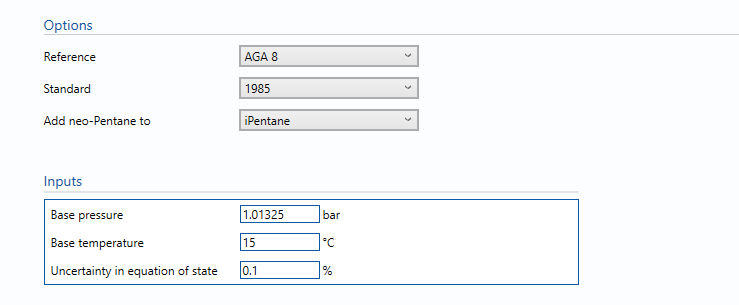#### Standard

• 1985
• 1994

This option determines which version of AGA 8 is used for the calculation of standard density.

• i-Pentane
• n-Pentane

As AGA 8 does not explicitly include neo-pentane this option is used to determine which component any neo-pentane included in the composition will be added to.

### Inputs

Base pressure

The pressure at which the standard density is to be calculated. This input is available if AGA 8 or ASTM D3588 is selected.

Base temperature

The temperature at which the standard density is to be calculated. This input is available if AGA 8 is selected.

U(EOS)

Uncertainty in the equation of state. Guidelines on determining this value can be found in the relevant standard.

### ASTM D3588

If ASTM D3588 is selected the following options will be available to the user: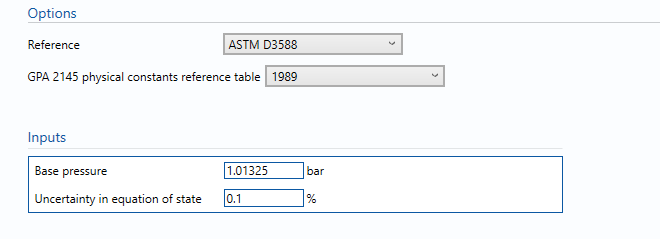#### GPA 2145 physical constants reference table

• 1989
• 2000
• 2003
• 2009

As physical properties data is periodically revised and updated this option is used to select which version of GPA 2145 will be used for the ASTM D3588 calculation.

### Inputs

Base pressure

The pressure at which the standard density is to be calculated. This input is available if AGA 8 or ASTM D3588 is selected.

U(EOS)

Uncertainty in the equation of state. Guidelines on determining this value can be found in the relevant standard.

### ISO 6976

If ISO 6976 is selected the following options will be available to the user: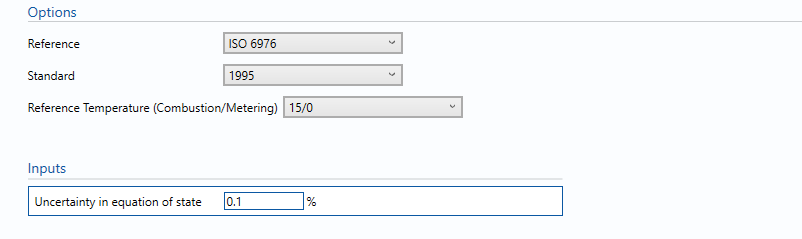#### Standard

• 1983
• 1989
• 1995

This option determines which version of ISO 6976 will be used for the calculation of standard density.

#### Reference Temperature (Combustion/Metering)

• 25/0
• 15/0
• 0/0
• 15/15
• 25/20 (only available if 1989 or 1995 is selected)
• 20/20 (only available if 1995 is selected)
• 25/15 (only available if 1995 is selected)

This option is used to select the standard temperature at which the density is calculated for. Note this is the second number and temperatures are in °C.

### Inputs

U(EOS)

Uncertainty in the equation of state. Guidelines on determining this value can be found in the relevant standard.

## Calculations

The gas standard density is calculated using the selected standard according to the option selected. The gas composition and uncertainty for each component are taken from the block B004 – Composition. The base pressure and base temperature will be taken according to the option selected or inputs given depending on which standard is being used.

### Uncertainty Budget

The uncertainty calculation is detailed in the uncertainty budget table giving a break down of how the overall uncertainty is calculated. The values input into the uncertainty budget are taken from calculations from other blocks along with the uncertainty in the equation of state as input in this block.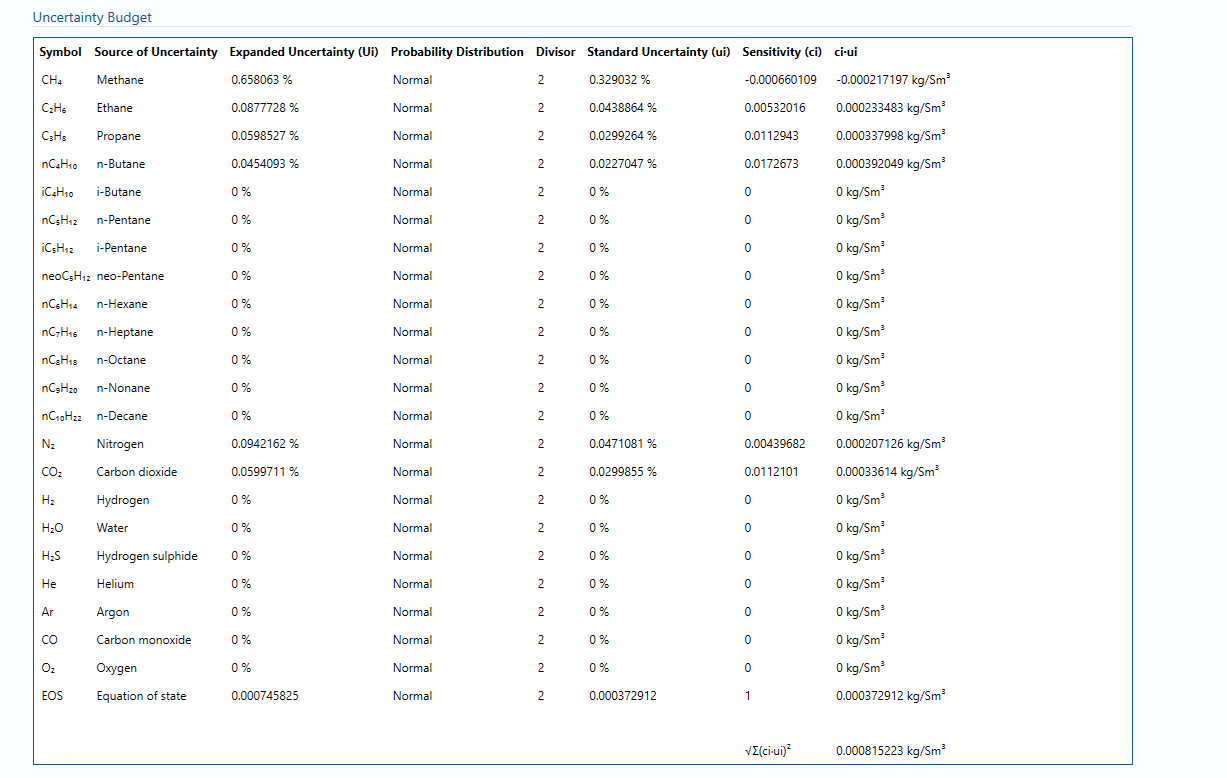Uncertainties associated with these values are taken in as the Expanded Uncertainties. The Standard Uncertainty is calculated from dividing the Expanded Uncertainty by the coverage factor which is determined by the probability distribution that best suits that uncertainty component.

The standard uncertainty is then multiplied by the sensitivity value then squared. This is done for each parameter that contributes to the overall uncertainty in gas standard density. The Standard Uncertainty in gas standard density is the square root of the sum of each component variance as shown in the following equation: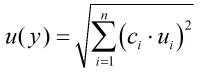Note: The sensitivity coefficients for each of these input uncertainties are determined using the finite difference method, where each of the calculation input quantities are varied, one at a time, by magnitude of their uncertainty, and the effect on the output determined.

### Calculated Uncertainty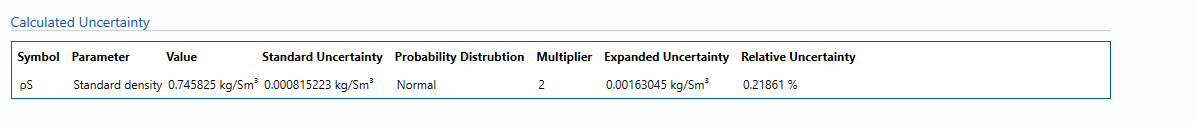The Expanded Uncertainty is the Standard Uncertainty multiplied by the coverage factor (k). The coverage factor is defaulted to k = 2 (equivalent to a confidence level of approximately 95%).

The Relative Uncertainty is the Expanded Uncertainty divided by the gas standard density value.

### Standards

ISO 5168:2005 – Measurement of fluid flow – Procedures for the evaluation of uncertainties

ISO Guide 98-3 – Uncertainty of measurement – Part 3: Guide to the expression of uncertainty in measurement (GUM:1995)

AGA Report No. 8 – Compressibility and Supercompressibility of Natural Gas and Other Related Hydrocarbon Gases (December 1985)

AGA Report No. 8 – Compressibility Factors of Natural Gas and Other Related Hydrocarbon Gases (2nd Printing July 1994)

ISO 12212-2:2006 – Natural Gas – Calculation of compression factor – Part 2: Calculation using molar composition analysis

ISO 6976:1983 – Natural gas – Calculation of calorific value, density and relative density

ISO 6976:1989 – Natural gas – Calculation of calorific value, density and relative density

ISO 6976:1995 – Natural gas – Calculation of calorific values, density, relative density and Wobbe index from composition

ASTM D3588-98: Standard Practice for Calculating Heat Value, Compressibility Factor and Relative Density of Gaseous Fuels (1998)

GPA 2145 Table of Physical Properties for Hydrocarbons and Other Compounds of Interest to the Natural Gas Industry (1989, 2000, 2003, 2009)

### KCCL Calculations

C111 – Calorific Value and Relative Density – ISO 6976

C156 – AGA8 1985/94 – Gas Density and Compressibility

C263 – ASTM D3588/GPA 2145 – Calorific Value and Relative Density

### Uncertainty Blocks

B004 – Composition

Back to Uncertainty Modules